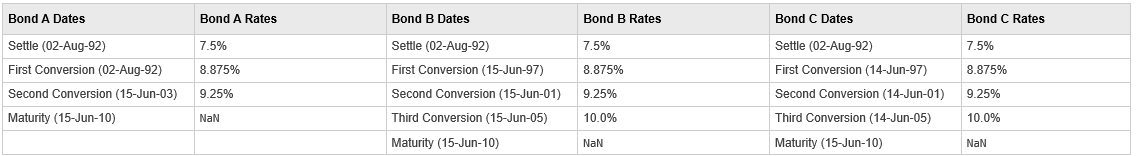stepcpnprice

Price bond with stepped coupons

Description

example

[Price,AccruedInterest] = stepcpnprice(Yield,Settle,Maturity,ConvDates,CouponRates) computes the price of bonds with stepped coupons given the yield to maturity. The function supports any number of conversion dates.

example

Examples

collapse all

Compute the price and accrued interest due on a portfolio of stepped-coupon bonds having a yield of 7.221%, given three conversion scenarios:

• Bond A has two conversions, the first one falling on the settle date and immediately expiring.

• Bond B has three conversions, with conversion dates exactly on the coupon dates.

• Bond C has three conversions, with one or more conversion dates not on coupon dates. This case illustrates that only cash flows for full periods after conversion dates are affected, as illustrated below:The following table illustrates the interest-rate characteristics of this bond portfolio.Define the specifications for the bonds.

Yield = 0.07221;
Settle   = datenum('02-Aug-1992');
ConvDates = [datenum('02-Aug-1992'), datenum('15-Jun-2003'),...
nan;
datenum('15-Jun-1997'), datenum('15-Jun-2001'),...
datenum('15-Jun-2005');
datenum('14-Jun-1997'), datenum('14-Jun-2001'),...
datenum('14-Jun-2005')];
Maturity = datenum('15-Jun-2010');

CouponRates = [0.075 0.08875 0.0925 nan;
0.075 0.08875 0.0925 0.1;
0.075 0.08875 0.0925 0.1];
Basis = 1;
Period = 2;
EndMonthRule = 1;
Face = 100;

Use stepcpnprice to compute the bond prices with stepped coupons.

[Price, AccruedInterest] = stepcpnprice(Yield, Settle, Maturity, ConvDates, CouponRates, Period, Basis, EndMonthRule, Face)
Price = 3×1

117.3874
113.4387
114.1759

AccruedInterest = 3×1

1.1587
0.9792
0.9792

Input Arguments

collapse all

Yield to maturity, specified as a scalar or NUMBONDS-by-1 vector of numeric values.

Data Types: double

Settlement date, specified either as a scalar or NUMBONDS-by-1 vector of serial date numbers.

Settle must be earlier than Maturity.

Data Types: double

Maturity date, specified as a scalar or an NUMBONDS-by-1 vector of serial date numbers representing the maturity date for each bond.

Data Types: double

Conversion dates, specified as a NSTP-by-max(NCONV) matrix containing conversion dates after Settle. The size of the matrix is equal to the number of instruments by the maximum number of conversions. Fill unspecified entries with NaN.

Data Types: double

Bond coupon rate, specified as an NSTP-by-max(NCONV+1) matrix containing coupon rates for each bond in the portfolio in decimal form. The matrix size is equal to the number of instruments by maximum number of conversions + 1. First column of this matrix contains rates applicable between Settle and the first conversion date (date in the first column of ConvDates). Fill unspecified entries with NaN

ConvDates has the same number of rows as CouponRates to reflect the same number of bonds. However, ConvDates has one less column than CouponRates. This situation is illustrated by

Settle---------ConvDate1-----------ConvDate2------------Maturity

Rate1               Rate2                 Rate3

Data Types: double

(Optional) Coupons per year, specified as an NUMBONDS-by-1 vector. Values for Period are 1, 2, 3, 4, 6, and 12.

Data Types: double

(Optional) Day-count basis of each instrument, specified as an NUMBONDS-by-1 vector.

• 0 = actual/actual

• 1 = 30/360 (SIA)

• 2 = actual/360

• 3 = actual/365

• 4 = 30/360 (PSA)

• 5 = 30/360 (ISDA)

• 6 = 30/360 (European)

• 7 = actual/365 (Japanese)

• 8 = actual/actual (ICMA)

• 9 = actual/360 (ICMA)

• 10 = actual/365 (ICMA)

• 11 = 30/360E (ICMA)

• 12 = actual/365 (ISDA)

• 13 = BUS/252

Data Types: double

(Optional) End-of-month rule flag for generating dates when Maturity is an end-of-month date for a month having 30 or fewer days, specified for each bond as a nonnegative integer [0, 1] using a NUMBONDS-by-1 vector.

• 0 = Ignore rule, meaning that a payment date is always the same numerical day of the month.

• 1 = Set rule on, meaning that a payment date is always the last actual day of the month.

Data Types: logical

(Optional) Face value, specified for each bond as an NUMBONDS-by-1 vector of nonnegative face values.

Data Types: double

Output Arguments

collapse all

Clean price, returned as a NUMBONDS-by-1 vector.

Note

For bonds with fixed coupons, use bndprice. If you use a fixed-coupon bond with stepcpnprice, you receive the error: incorrect number of inputs.

accrued interest payable at settlement dates, returned as a NUMBONDS-by-1 vector.

Introduced before R2006a